# Balayage method

A method for solving the Dirichlet problem for the Laplace equation, developed by H. Poincaré (, , see also ), which will now be described. Letbe a bounded domain of the Euclidean space,, let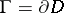be the boundary of. Let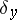be the Dirac measure concentrated at the point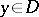, letbe the Newton potential of the measurefor, or the logarithmic potential of the measureif. A balayage (or sweeping) of the measurefrom the domainto the boundaryis a measure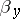onwhose potential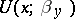coincides outsidewith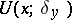and is not larger thaninside; this measureis unique and coincides with the harmonic measure onfor the point. The balayage of an arbitrary positive measure, concentrated on, is defined in a similar manner. Ifis a sphere, the density of the mass distribution, i.e. the derivative of the measure, is identical with the Poisson kernel (cf. Poisson integral). In general, if the boundaryis sufficiently smooth, the measureis absolutely continuous, and the density of the mass distributioncoincides with the normal derivative of the Green function for. The measureserves to write down the solution of the Dirichlet problem as the so-called formula of de la Vallée-Poussin:where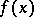is a function defined on.

In his original publication on the balayage method, Poincaré began by demonstrating the geometrical construction of the process for a sphere. Then, basing himself on Harnack's theorems (cf. Harnack theorem) and on the fact that it is possible to exhaust the domainby a sequence of spheres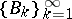, he constructed an infinite sequence of potentials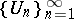in which each potential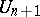is obtained from the preceding one,, by the balayage method of moving the masses from the domain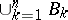to its boundary, and which reduces to solving the Dirichlet problem for a sufficiently smooth domain(for a detailed discussion of the conditions of applicability of the balayage method, see ).

In modern potential theory ,  the balayage problem is treated as an independent problem, resembling the Dirichlet problem, and it turns out that the balayaged measure can be considered on sets of a general nature. For instance, the balayage problem in its simplest form is to find, for a given mass distributioninside a closed domain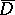, a mass distributiononsuch that the potentials of both distributions coincide outside. If the boundaryis smooth, the solution of the balayage problem forwill be an absolutely continuous measure. Its density, or the derivative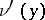,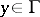, may be written down in terms of the Green function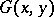of the domainin the form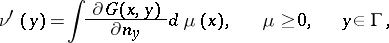(*)

where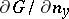is the derivative ofin the direction of the interior normal toat the point. Inside the domainthe potentials satisfy the inequality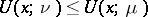, i.e. balayage inside the domain results in a decrease of the potential. Ifis the Dirac measure at the point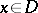, formula (*) yields, i.e. the normal derivative of the Green function is the density of the measure obtained by balayage of the unit mass concentrated at the point. Generalization of formula (*) yields an expression for the balayaged measure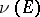of an arbitrary Borel setfor an arbitrary domain: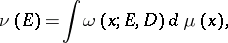where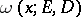is the harmonic measure ofwith respect to the domainat the point.

Ifis an arbitrary compact set in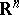andis a bounded positive Borel measure, the balayage (or sweeping) of the measureonto the compact setis a measureonsuch thateverywhere, and such that quasi-everywhere on, i.e. with the possible exception of a set of points of exterior capacity zero,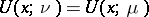. Such a formulation of the balayage problem, which is more general than balayage from a domain, may also be extended to potentials of other types, e.g. Bessel potentials or Riesz potentials (cf. Bessel potential; Riesz potential). Balayage of measures onto arbitrary Borel setsis also considered.

The problem of balayage for superharmonic functions (cf. Superharmonic function) has been similarly formulated. Let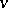be a non-negative superharmonic function on a domain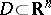. The balayage of the functiononto a compact setis the largest superharmonic functionsuch that 1) its associated measure is concentrated on; 2)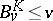everywhere; and 3)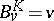quasi-everywhere on.

In abstract potential theory (cf. Potential theory, abstract) the balayage problem in both its formulations is solved for setsin an arbitrary harmonic space, i.e. in a locally compact topological spacewhich permits the isolation of an axiomatically defined sheaf of harmonic functions. This axiomatic approach makes it possible to consider the balayage problem for potentials connected with partial differential equations of a more general nature . For the balayage method in stochastics cf. .